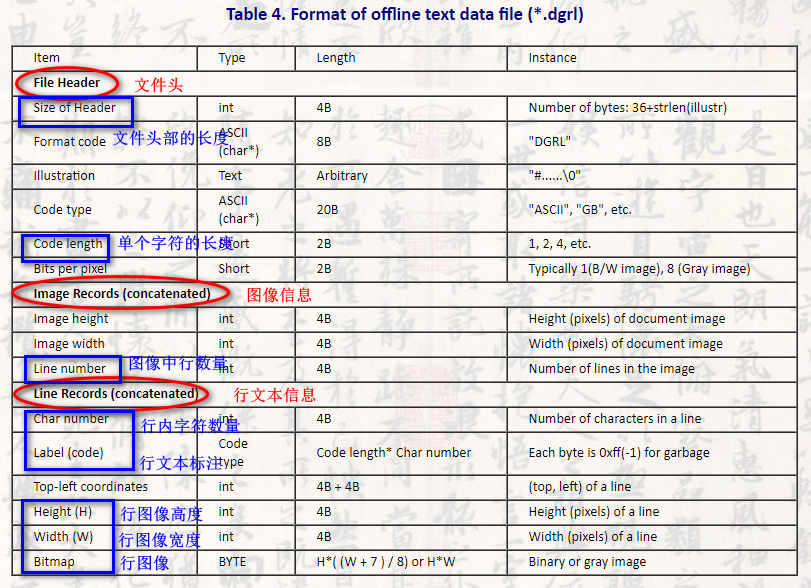# CASIA-HWDB2.x 数据集DGRL文件解析（python）

CASIA-HWDB 数据集是最常见的手写汉字识别数据集，它包含脱机、联机两部分，分单字、文本行两种类型：

• HWDB1.x：脱机单字，1.0~1.2 三个版本，数据格式为 .gnt
• OLHWDB1.x：联机单字，1.0~1.2 三个版本，
• HWDB2.x：脱机文本行，1.0~1.2 三个版本，数据格式为 .dgrl
• OLHWDB1.x：联机文本行，1.0~1.2 三个版本，

##### 1、DGRL 文件格式介绍

.dgrl 格式如下图所示，它按照这种顺序依次存储，一张图对应一个 DGRL 文件，大部分内容都有固定的长度，部分内容长度不固定但是也能通过其他数据推导出来，我们可以通过访问文件特定位置的数据得到我们需要的内容：行文本标注，行图像。##### 2、python 代码实现

f = open(dgrl, 'rb')


np.fromfile(f, dtype='uint8', count=4)


struct.pack('I', i).decode('gbk', 'ignore')


import struct
import os
import cv2 as cv
import numpy as np

if not os.path.exists(dgrl):
print('DGRL not exis!')
return

dir_name,base_name = os.path.split(dgrl)
label_dir = dir_name+'_label'
image_dir = dir_name+'_images'
if not os.path.exists(label_dir):
os.makedirs(label_dir)
if not os.path.exists(image_dir):
os.makedirs(image_dir)

with open(dgrl, 'rb') as f:
# 读取表头尺寸

# 读取表头剩下内容，提取 code_length
code_length = sum([j<<(i*8) for i,j in enumerate(header[-4:-2])])
# print(code_length)

# 读取图像尺寸信息，提取图像中行数量
image_record = np.fromfile(f, dtype='uint8', count=12)
height = sum([j<<(i*8) for i,j in enumerate(image_record[:4])])
width = sum([j<<(i*8) for i,j in enumerate(image_record[4:8])])
line_num = sum([j<<(i*8) for i,j in enumerate(image_record[8:])])
print('图像尺寸:')
print(height, width, line_num)

# 读取每一行的信息
for k in range(line_num):
print(k+1)

# 读取该行的字符数量
char_num = np.fromfile(f, dtype='uint8', count=4)
char_num = sum([j<<(i*8) for i,j in enumerate(char_num)])
print('字符数量:', char_num)

# 读取该行的标注信息
label = np.fromfile(f, dtype='uint8', count=code_length*char_num)
label = [label[i]<<(8*(i%code_length)) for i in range(code_length*char_num)]
label = [sum(label[i*code_length:(i+1)*code_length]) for i in range(char_num)]
label = [struct.pack('I', i).decode('gbk', 'ignore') for i in label]
print('合并前：', label)
label = ''.join(label)
label = ''.join(label.split(b'\x00'.decode()))	# 去掉不可见字符 \x00，这一步不加的话后面保存的内容会出现看不见的问题
print('合并后：', label)

# 读取该行的位置和尺寸
pos_size = np.fromfile(f, dtype='uint8', count=16)
y = sum([j<<(i*8) for i,j in enumerate(pos_size[:4])])
x = sum([j<<(i*8) for i,j in enumerate(pos_size[4:8])])
h = sum([j<<(i*8) for i,j in enumerate(pos_size[8:12])])
w = sum([j<<(i*8) for i,j in enumerate(pos_size[12:])])
# print(x, y, w, h)

# 读取该行的图片
bitmap = np.fromfile(f, dtype='uint8', count=h*w)
bitmap = np.array(bitmap).reshape(h, w)

# 保存信息
label_file = os.path.join(label_dir, base_name.replace('.dgrl', '_'+str(k)+'.txt'))
with open(label_file, 'w') as f1:
f1.write(label)
bitmap_file = os.path.join(image_dir, base_name.replace('.dgrl', '_'+str(k)+'.jpg'))
cv.imwrite(bitmap_file, bitmap)


01-07378

#### 【OCR炼丹】解析CASIA数据集OLHWDB部分Python版完整代码12-316784

#### CASIA中文手写体字库gnt文件格式解析(python)

01-021524

#### 基于caffe和casiaHWDB手写体汉字库的LMDB数据准备

12-06385

#### 如何使用CASIA-OLHWDB联机手写汉字数据集？（解答篇）©️2020 CSDN 皮肤主题: 大白 设计师: CSDN官方博客点击重新获取扫码支付1.余额是钱包充值的虚拟货币，按照1:1的比例进行支付金额的抵扣。
2.余额无法直接购买下载，可以购买VIP、C币套餐、付费专栏及课程。余额充值